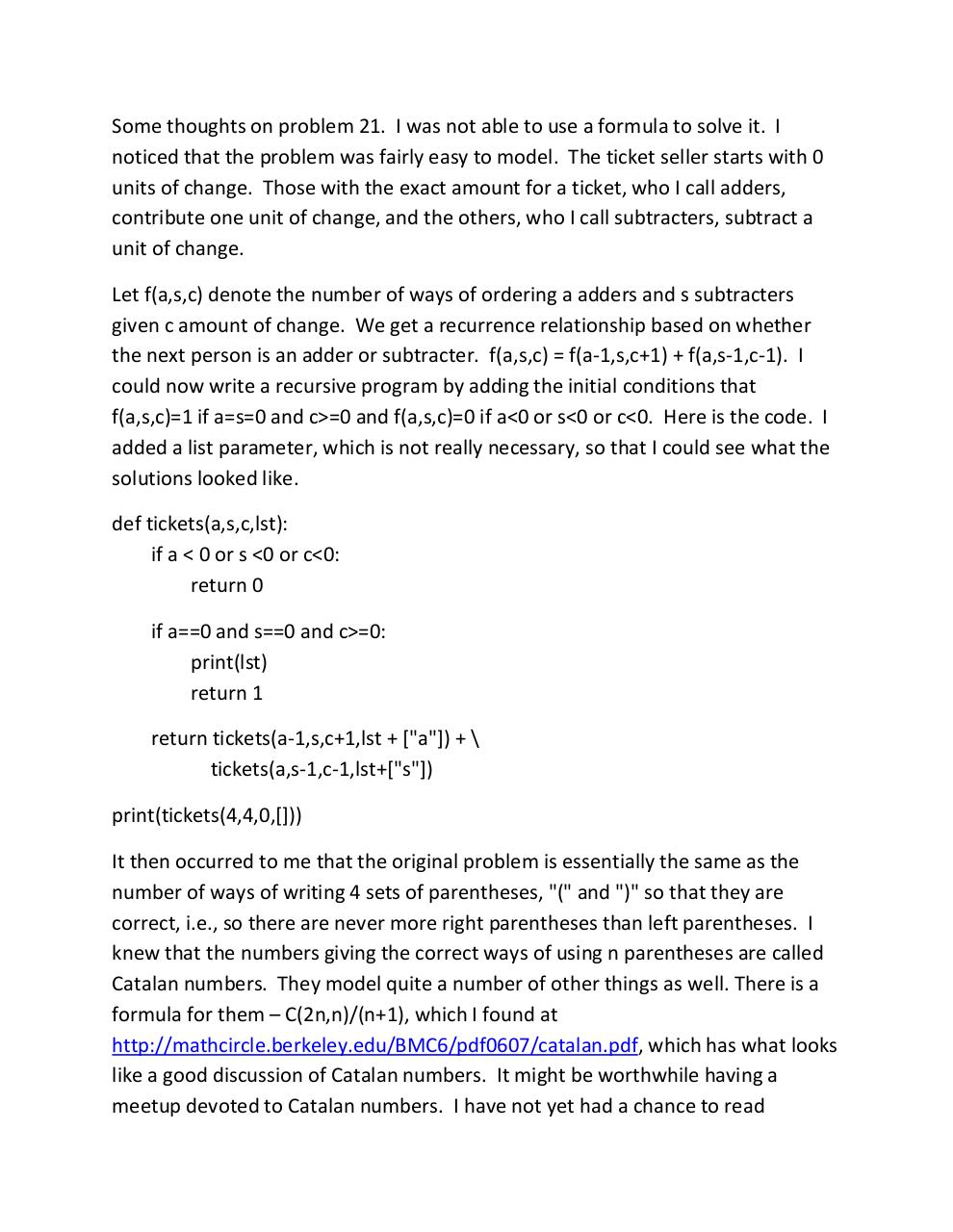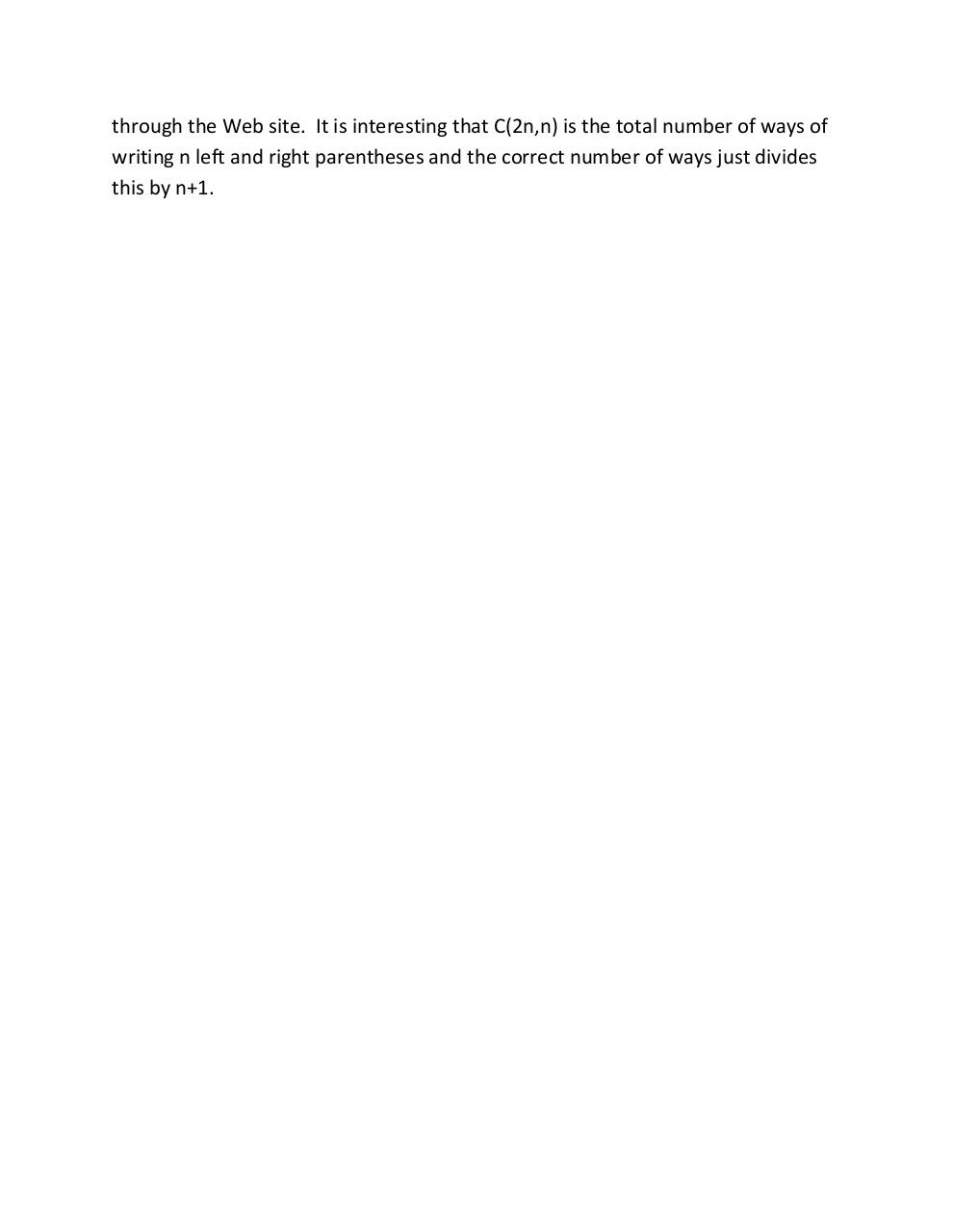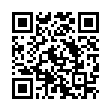# SomeThoughtsOnProblem21 .pdf

### File information

Original filename: SomeThoughtsOnProblem21 .pdf
Author: Martin Cohen

This PDF 1.5 document has been generated by MicrosoftÂ® Word Starter 2010, and has been sent on pdf-archive.com on 01/03/2017 at 21:11, from IP address 74.116.x.x. The current document download page has been viewed 226 times.
File size: 120 KB (2 pages).
Privacy: public file

SomeThoughtsOnProblem21 .pdf (PDF, 120 KB)

### Document preview

Some thoughts on problem 21. I was not able to use a formula to solve it. I
noticed that the problem was fairly easy to model. The ticket seller starts with 0
units of change. Those with the exact amount for a ticket, who I call adders,
contribute one unit of change, and the others, who I call subtracters, subtract a
unit of change.
Let f(a,s,c) denote the number of ways of ordering a adders and s subtracters
given c amount of change. We get a recurrence relationship based on whether
the next person is an adder or subtracter. f(a,s,c) = f(a-1,s,c+1) + f(a,s-1,c-1). I
could now write a recursive program by adding the initial conditions that
f(a,s,c)=1 if a=s=0 and c&gt;=0 and f(a,s,c)=0 if a&lt;0 or s&lt;0 or c&lt;0. Here is the code. I
added a list parameter, which is not really necessary, so that I could see what the
solutions looked like.
def tickets(a,s,c,lst):
if a &lt; 0 or s &lt;0 or c&lt;0:
return 0
if a==0 and s==0 and c&gt;=0:
print(lst)
return 1
return tickets(a-1,s,c+1,lst + ["a"]) + \
tickets(a,s-1,c-1,lst+["s"])
print(tickets(4,4,0,[]))
It then occurred to me that the original problem is essentially the same as the
number of ways of writing 4 sets of parentheses, "(" and ")" so that they are
correct, i.e., so there are never more right parentheses than left parentheses. I
knew that the numbers giving the correct ways of using n parentheses are called
Catalan numbers. They model quite a number of other things as well. There is a
formula for them – C(2n,n)/(n+1), which I found at
http://mathcircle.berkeley.edu/BMC6/pdf0607/catalan.pdf, which has what looks
like a good discussion of Catalan numbers. It might be worthwhile having a
meetup devoted to Catalan numbers. I have not yet had a chance to read

through the Web site. It is interesting that C(2n,n) is the total number of ways of
writing n left and right parentheses and the correct number of ways just divides
this by n+1.#### HTML Code

Copy the following HTML code to share your document on a Website or Blog

#### QR Code### Related keywords